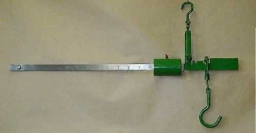# Equilibrium 7990

At the end of one arm of the equilibrium scales, which are in equilibrium, a lead body with a volume V1 is suspended in the air. At the end of the other arm is an aluminum body with a volume V2. The balance arms have sizes l1 and l2, lead density h1 = 11,340 kg / m3, aluminum density h2 = 2,700 kg / m3. If we change the position of the bodies and immerse them in water, equilibrium occurs again (h3 = 1000 kg / m3). Do not consider the buoyancy of the air.

a) What is the ratio of arm sizes l1: l2?
b) What is the volume ratio of V2: V1 bodies?

a =  1.2034
b =  5.0543

### Step-by-step explanation:

Our quadratic equation calculator calculates it.Did you find an error or inaccuracy? Feel free to write us. Thank you!

Tips to related online calculators
Looking for help with calculating roots of a quadratic equation?
Do you have a linear equation or system of equations and looking for its solution? Or do you have a quadratic equation?
Tip: Our volume units converter will help you with the conversion of volume units.
Tip: Our Density units converter will help you with the conversion of density units.